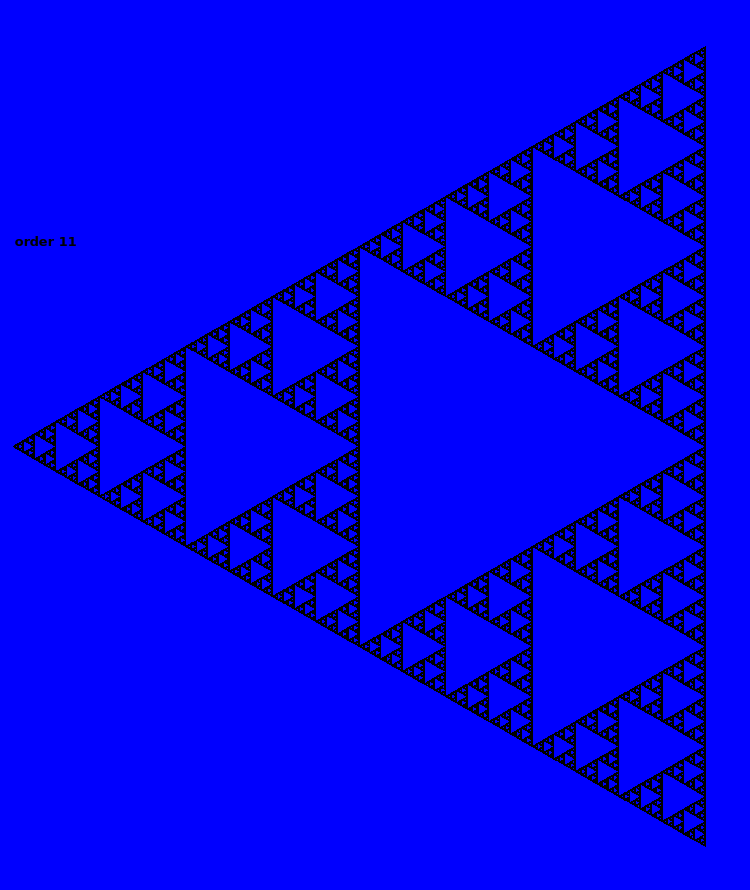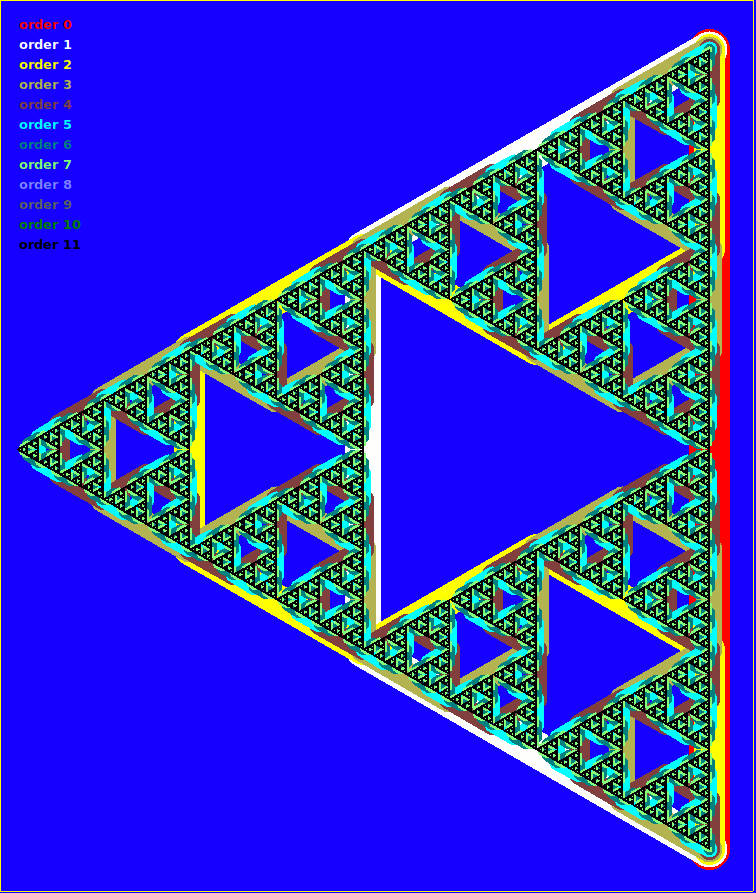# Sierpinski curves-## Code

Code- one of many versions.

You specify the axiom and rule and angle, and it iteratively creates the long string of moves to be drawn,

```    'QO fractal generator version f

nomainwin

UpperLeftX   =  10
UpperLeftY   =   2
WindowWidth  =1020
WindowHeight = 730

textbox    #w.text,      2,   2,1000,  30
graphicbox #w.graphic,   2,  40,1000, 550
texteditor #w.tedit,     2, 600,1000, 200

button     #w.b, "Draw", [doit], UL, 930, 50, 50, 30

open "QO Fractal Generator" for window_nf as #w

#w         "trapclose quit"
#w.graphic "size 2 ; color black ; cls ; fill darkblue"
#w         "font courier_new 8"

'axiom\$    =       "F"
axiom\$    =        "F"
rule\$     =       "F-F++F-F"
'rule\$     =        "F-F+F+F-F"
n         =        0
pi        =        4 *atn( 1)
angle     =       60 *pi /180
'angle     =       90 *pi /180

[doit]
n =n +1
if n >7 then call quit h\$

totalx    =        0
totaly    =        0
newrule\$  =       ""
direction =        0    '   NB in RADIANS

state =1

#w.tedit "Iteration # ";
#w.tedit  n;
#w.tedit " & rule = "

l   =len( axiom\$)

for k =1 to l /128
#w.tedit mid\$( axiom\$, k, 128)
next k

for i =1 to len( axiom\$)
ch\$ =mid\$( axiom\$, i, 1)

select case ch\$
case "+"
direction =direction +angle
newrule\$  =newrule\$  +"+"
case "-"
direction =direction -angle
newrule\$  =newrule\$  +"-"
case "F"
newrule\$ =newrule\$ +rule\$
totalx   = totalx +cos( direction)
totaly   = totaly +sin( direction)
end select
next i

op\$ ="Total x = " +str\$( int( totalx)) +"   & total y = " +str\$( int( totaly))
#w.text op\$

ste =440 /totalx
xx =10: yy =250: direction =0

#w.graphic "cls ; fill darkblue"
#w.graphic "up ; goto  20 500 ; down ; north; turn 90"
'#w.graphic "color "; str\$( 40 +30 *n); " "; str\$( 40 +30 *n); " 50"
'if state =1 then state =0 else state =1
#w.graphic "color "; word\$( "black white", state +1)
#w.graphic "size "; 1 +2 *( 7 -n)

for i =1 to len( axiom\$)

in\$ =mid\$( axiom\$, i, 1)

select case in\$
case "F"
xx =xx +ste *cos( direction)
yy =yy +ste *sin( direction)
case "+"
direction =direction +angle
case "-"
direction =direction -angle
end select

#w.graphic "goto "; str\$( int( 2 *xx)); " "; str\$( 2 *int( yy))

scan

#w.graphic "flush ; getbmp scr 0 0 1000 550"
bmpsave "scr", "IFSrule" +rule\$ +str\$( n) +".bmp"
next i

axiom\$ =newrule\$

timer 1000, [o]
wait
[o]
timer 0
wait

sub quit h\$
close #w
end
end sub
```

We've had several visits to this, including for Rosetta Code. There are three variations that produce the same figure but in very different ways.

Draw scaled and moved triangles

Do by IFS ( iterated file function system)

Draw as a single non-imtersecting line curve.

While I've coded all ways, this is the latter one. Was writing in LB5 on Linux and found one or two glitches.

I was particularly pleased by the graphic created by overlaying each iteration in a different colour and with thinner lines to match the greater resolution. Worth watching it ruin!

## Code

```'   ***********************************************************************
'   **                                                                   **
'   **    sierpinskiArrow2alteredHoriz.bas   tenochtitlanuk   24/06/30   **
'   **                                                                   **
'   ***********************************************************************

nomainwin

global pi, TX, TY, Ttheta

TX =400: TY =350: Ttheta =0 '   screen centre, pointing North/up.   '   <<<<<<<<<<<<<<<<<<<<<<<<<
pi =4 *atn( 1)

WindowWidth  = 760
WindowHeight = 900

open "Sierpinski arrowhead" for graphics_nsb as #wg

#wg "trapclose quit"
#wg "color cyan ; font Arial bold 18"
#wg "cls ; down ; fill blue ; backcolor blue ; flush"

'width =11

for i =0 to 11
'#wg "cls ; down ; fill blue ; backcolor blue ; flush"   '   rem this line to see each iteration on its own...
#wg  "color "; word\$( "red,white,yellow,180 180 80,brown,cyan,darkcyan,130 255 120,131 130 255,darkgray,darkgreen,darkpink,darkred,green,lightgray,palegray,pink,blue", i +1, ",")
#wg "up ; goto 20 "; 30 +20 * i; " ; down"
#wg "\order "; i
#wg "size "; max( 1, 41 -5 *i)
TX =710: TY =850: Ttheta =-90
call SierpinskiArrowhead i, 800 '   order, length
#wg "flush"
#wg "getbmp scr 0 0 1400 900"
'bmpsave "scr", "scr3/SierpinskiArrow" +str\$( i) +".bmp"
call Sleep 2000
next i
wait
sub quit h\$
close #wg
end
end sub

sub Sleep ms
timer ms, [k]
wait
[k]
timer 0
end sub

if ( order and 1) =0 then    ' order is even..
call curve order, length, 60
else
call turn -60
call curve order, length, 60
end if
end sub

sub curve order, length, angle
scan
if order =0 then
'#wg "go "; length
call forward length
else
'#wg "turn " +str\$( angle)
call curve order -1, length /2, 0 -angle
call turn angle
call curve order -1, length /2, 0 +angle
'#wg "turn " +str\$( angle)
call turn angle
call curve order -1, length /2, 0 -angle
end if
end sub

'   <<<<<<<<<<<<<<<<<<<<<<<<<
end function

end function

sub draw lifted, x, y
if lifted =0 then #wg "up" else #wg "down"
#wg "line "; TX; " "; TY; " "; x; " "; y
Ttheta  =atan2( x -TX, TY -y) *180 /pi  '   NB DEGREES.
TX      =x
TY      =y
end sub

sub turn angle  '   increment/update global turtle direction ( in DEGREES)
Ttheta =( Ttheta +angle)
if Theta <0 then Ttheta =Ttheta +360
Ttheta =Ttheta mod 360
end sub

sub forward s
#wg "down ; line "; TX; " "; TY; " "; TX +dx; " "; TY +dy; " ; up"
TX =TX +dx
TY =TY +dy
end sub

function atan2( x, y)
Result\$ = "Undetermined"
If ( x = 0) and ( y > 0) Then atan2 = pi / 2:     Result\$ = "Determined"
If ( x = 0) and ( y < 0) Then atan2 = 3 * pi / 2: Result\$ = "Determined"
If ( x > 0) and ( y = 0) Then atan2 = 0:          Result\$ = "Determined"
If ( x < 0) and ( y = 0) Then atan2 = pi:         Result\$ = "Determined"
If Result\$ = "Determined" Then [End.of.function]
BaseAngle = Atn( abs( y) /abs( x))
If (x > 0) and (y > 0) Then atan2 =        BaseAngle
If (x < 0) and (y > 0) Then atan2 = pi    -BaseAngle
If (x < 0) and (y < 0) Then atan2 = pi    +BaseAngle
If (x > 0) and (y < 0) Then atan2 = 2*pi  -BaseAngle
[End.of.function]
end function
'   <<<<<<<<<<<<<<<<<<<<<<<<
```